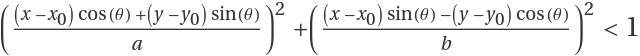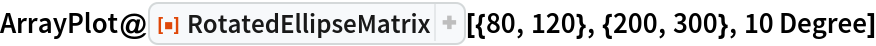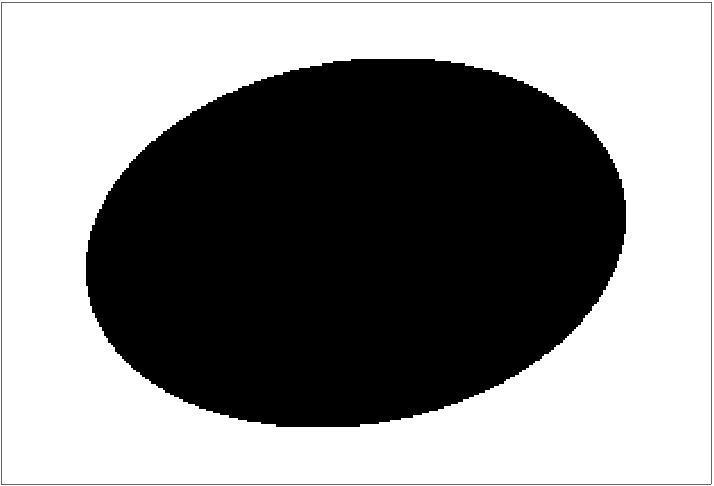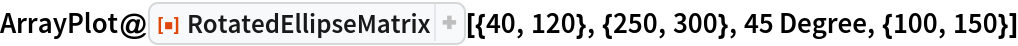Function Repository Resource:

# RotatedEllipseMatrix

Create a binary matrix with a rotated, ellipse-shaped region of 1s

Contributed by: Štěpán Venos
 ResourceFunction["RotatedEllipseMatrix"][{a,b},{m,n}] gives an m×n matrix whose elements are 1 in a centered, ellipse-shaped region of axis lengths a and b, and are otherwise 0. ResourceFunction["RotatedEllipseMatrix"][{a,b},{m,n},θ] gives an ellipse-shaped region rotated by θ radians. ResourceFunction["RotatedEllipseMatrix"][{a,b},{m,n},θ,{x0,y0}] gives an ellipse-shaped region rotated by θ radians and with center {x0,y0}.

## Details and Options

The equation of a rotated ellipse region is.

## Examples

### Basic Examples (1)

Generate an array plot of an ellipse matrix:

 In:=Out=### Scope (1)

Generate an array plot of an off-centered ellipse matrix:

 In:=Out=Stepan Venos

## Version History

• 1.0.0 – 06 August 2019